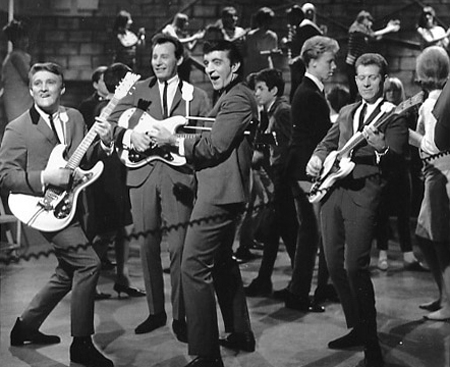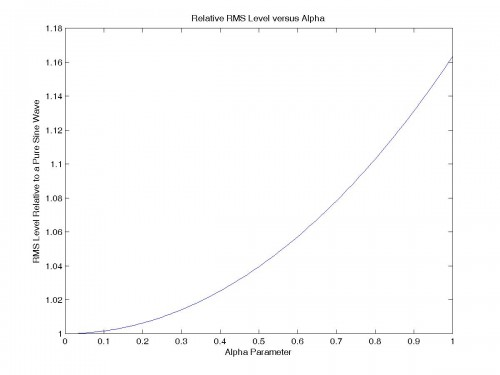## Euphonic Distortion?Why is it that every time you mention that a piece of audio equipment sounds better than another, the spectre of “euphonic distortion” is invoked?  Is it possible that it is really that simple?  You just add some 2nd harmonic distortion and poof!  Now it sounds better?

Enough hand waving already.  They say low order harmonic distortion makes it sound better, I say it doesn’t.  Who’s right?  Well everybody prepare yourselves because…That’s right, science!  And there’s nothing you can do to stop me – mwaah ha ha ha!

First off let’s start simple.  Some folks say it sounds better when you add low order harmonic distortion.  One of these words in particular stands out to me: “add”.  Why does this word in particular jump out?  It’s due to the importance of level matching.  Most audiophiles know how critical it is to accurately match audio equipment if there’s any hope to perform a fair comparison, but it seems many people have forgotten in this one area.

If you add distortion, then you must adjust the level so that the RMS level matches that of the original signal!

As a concrete example, let’s consider a very simple audio amplifier: A single bipolar junction transistor.  Here’s an outline of the process to follow:

• Determine the appropriate function of the distortion mechanism
• Determine the average value in order to remove any dc component
• Determine the RMS value and apply a multiplier to compensate

Here is the equation for the single bipolar transistor stage when passing a sine wave of angular frequency$\omega$:$f(t)=\frac{1}{\alpha }\exp(\alpha \sin(\omega t))$

Where$\alpha$ is the degree of nonlinearity – i.e. lower distortion for smaller$\alpha$ and higher distortion for larger$\alpha$.

Integrating over a full cycle to get the average (i.e. dc) value:$f_{AVG}=\frac{1}{2\pi }\int_{0}^{2\pi }\frac{1}{\alpha }\exp(\alpha \sin(x))dx=\frac{1}{\alpha}I_{0}(\alpha)$

Where$I_{0}(\alpha)$ is a modified Bessel function of the first kind.  Now subtract this dc component from the original equation for the transistor stage to get the ac value:$f_{AC}(t)=\frac{1}{\alpha }(\exp(\alpha \sin(\omega t))-I_{0}(\alpha))$

From this the RMS value can now be calculated.  Here is a general expression for calculating the RMS value of a function:$f_{RMS}=\left [ \frac{1}{t_{2}-t_{1}}\int_{t_{1}}^{t_{2}} f^{2}(t)dt \right ]^{1/2}$

Now using this to calculate the RMS value of the single stage bipolar transistor when passing a sine wave:$f_{RMS}=\left [ \frac{1}{2\pi }\int_{0}^{2\pi}f_{AC}^{2}(t)dt \right ]^{1/2}=\frac{1}{\alpha}\left [I_{0}(2\alpha)-I_{0}^{2}(\alpha) \right ]^{1/2}$

Here’s a plot of this$f_{RMS}$ function versus the distortion parameter$\alpha$:So now to make the RMS level of the distorted sine wave equal to that of the undistorted sine wave, you must multiply the amplitude of the pure sine wave by the above$f_{RMS}$ expression.  You could instead divide the distorted sine wave by the same value – the point is to make the two RMS levels equal.

For clarity here are the two expressions with differing distortion, but equal RMS level – again$\alpha$ is the distortion parameter (distortion → 0 as$\alpha$ → 0):$f_{UNDISTORTED}(t)=\frac{1}{\alpha}\left [I_{0}(2\alpha)-I_{0}^{2}(\alpha) \right ]^{1/2} \sin(\omega t)$$f_{DISTORTED}(t)=\frac{1}{\alpha }(\exp(\alpha \sin(\omega t))-I_{0}(\alpha))$

As a further point of interest, the nature of the distortion mechanism considered above is that of a nice descending spectrum of harmonics, as shown in the following FFT plot for$\alpha$=0.1:I’m still doing a bit more work with this, so it is by no means anywhere close to conclusive.  However, it is at least an important, and objective, difference that must be accounted for in order to compare “apples to apples” when evaluating the subjective effect of different distortion mechanisms.